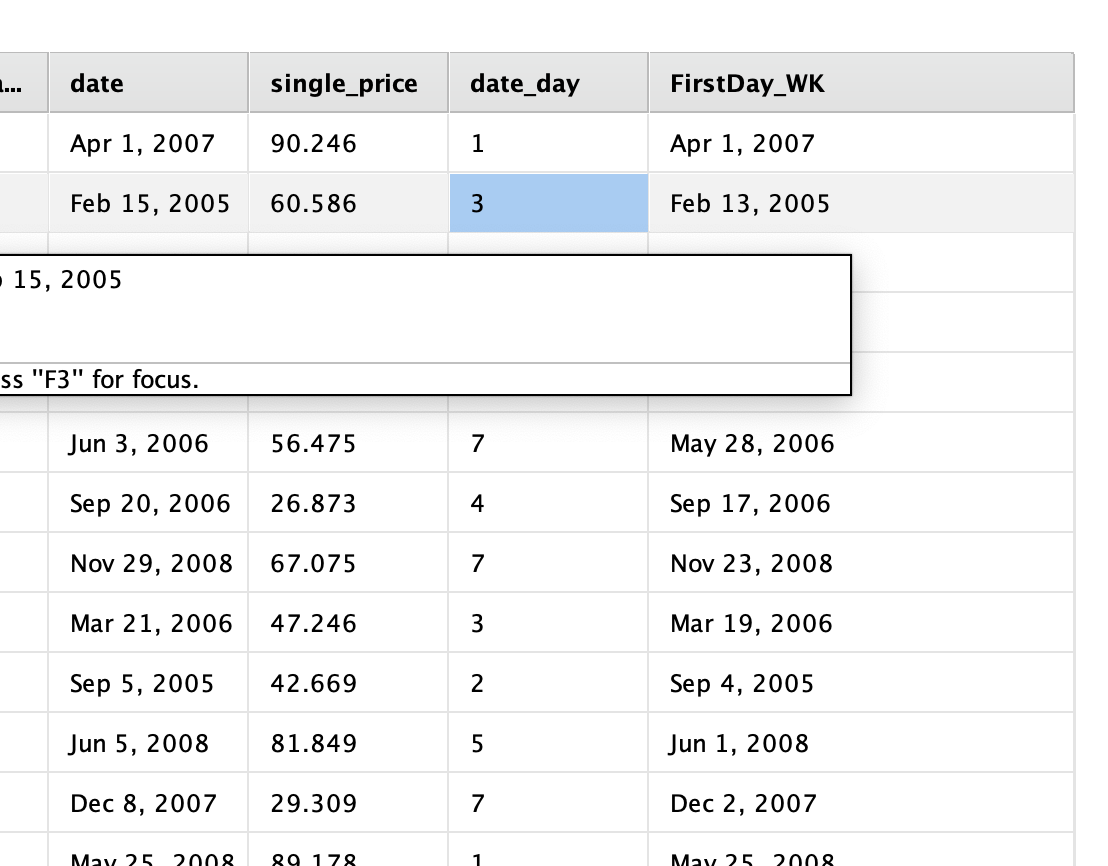# First day of each week

Member Posts: 35Contributor II
Hello,
I want to do a time series forecast in data that I wish to group by week and I use PIVOT to do that. The problem is that then I lose the date type and I don't want it. Before Rapidminer I use to use Excel to transform year and week numbers in date (as first day of each week). By doing this I get a monotonic time series.

In Excel I used  to calculate the first day of each week like below, but I want to perform all data prep in RM and honestly I'm getting nowhere.

=DATE([@YEAR],1,-2)-WEEKDAY(DATE([@YEAR],1,3))+[@WEEK]*7

I bet RM can do this easily with generate attributes operator, but I'm totally stucked.

Pedro
Tagged:

• Administrator, Moderator, Employee, RapidMiner Certified Analyst, RapidMiner Certified Expert, Community Manager, RMResearcher, Member, University Professor Posts: 1,751RM Founder
Ok, that formula does not work in Excel for me :-)  Can you post a quick data example, i.e. the original dates and what the result of the extracted column should look like?  That may help since somehow I struggle to follow along.
Cheers,
Ingo
• Administrator, Employee, RapidMiner Certified Analyst, RapidMiner Certified Expert, Member Posts: 363RM Data Scientist
edited March 2019
Hi @Oprick,

It is a little different that Sunday is usually the starting of a week in USA. But if you need the first day of the week to be defined as Monday, it is a simple fix.

My process use "date to numerical" to extract the day relative to week first. If the date is already Sunday (day 1), I will keep it the same. Otherwise, the formula will generate a new date:```<?xml version="1.0" encoding="UTF-8"?><process version="9.2.000">
<context>
<input/>
<output/>
<macros/>
</context>
<operator activated="true" class="process" compatibility="9.2.000" expanded="true" name="Process">
<parameter key="logverbosity" value="init"/>
<parameter key="random_seed" value="2001"/>
<parameter key="send_mail" value="never"/>
<parameter key="process_duration_for_mail" value="30"/>
<parameter key="encoding" value="SYSTEM"/>
<process expanded="true">
<operator activated="true" class="generate_sales_data" compatibility="9.2.000" expanded="true" height="68" name="Generate Sales Data" width="90" x="112" y="34">
<parameter key="number_examples" value="100"/>
<parameter key="use_local_random_seed" value="false"/>
<parameter key="local_random_seed" value="1992"/>
</operator>
<operator activated="true" class="date_to_numerical" compatibility="9.2.000" expanded="true" height="82" name="Date to Numerical" width="90" x="313" y="34">
<parameter key="attribute_name" value="date"/>
<parameter key="time_unit" value="day"/>
<parameter key="millisecond_relative_to" value="second"/>
<parameter key="second_relative_to" value="minute"/>
<parameter key="minute_relative_to" value="hour"/>
<parameter key="hour_relative_to" value="day"/>
<parameter key="day_relative_to" value="week"/>
<parameter key="week_relative_to" value="year"/>
<parameter key="month_relative_to" value="year"/>
<parameter key="quarter_relative_to" value="year"/>
<parameter key="half_year_relative_to" value="year"/>
<parameter key="year_relative_to" value="era"/>
<parameter key="keep_old_attribute" value="true"/>
</operator>
<operator activated="true" class="generate_attributes" compatibility="9.2.000" expanded="true" height="82" name="Generate Attributes" width="90" x="514" y="34">
<list key="function_descriptions">
</list>
<parameter key="keep_all" value="true"/>
</operator>
<operator activated="true" class="date_to_nominal" compatibility="9.2.000" expanded="true" height="82" name="Date to Nominal" width="90" x="648" y="34">
<parameter key="attribute_name" value="FirstDay_WK"/>
<parameter key="date_format" value="MMM d, yyyy"/>
<parameter key="time_zone" value="SYSTEM"/>
<parameter key="locale" value="English (United States)"/>
<parameter key="keep_old_attribute" value="false"/>
</operator>
<operator activated="false" class="nominal_to_date" compatibility="9.2.000" expanded="true" height="82" name="Nominal to Date" width="90" x="514" y="136">
<parameter key="attribute_name" value=""/>
<parameter key="date_type" value="date"/>
<parameter key="date_format" value="MMM d, yyyy"/>
<parameter key="time_zone" value="SYSTEM"/>
<parameter key="locale" value="English (United States)"/>
<parameter key="keep_old_attribute" value="false"/>
</operator>
<connect from_op="Generate Sales Data" from_port="output" to_op="Date to Numerical" to_port="example set input"/>
<connect from_op="Date to Numerical" from_port="example set output" to_op="Generate Attributes" to_port="example set input"/>
<connect from_op="Generate Attributes" from_port="example set output" to_op="Date to Nominal" to_port="example set input"/>
<connect from_op="Date to Nominal" from_port="example set output" to_port="result 1"/>
<portSpacing port="source_input 1" spacing="0"/>
<portSpacing port="sink_result 1" spacing="0"/>
<portSpacing port="sink_result 2" spacing="0"/>
</process>
</operator>
</process>
```

• Member Posts: 35Contributor II
Hi,
@IngoRM I believe that is due to different date settings, but what the formula does is the following.
DATE is built using YEAR and WEEK and Date is the first day of the corresponding week and year.

 YEAR WEEK DATE 2019 10 03/04/2019 2019 9 02/25/2019 2019 8 02/18/2019 2019 8 02/18/2019

@yyhuang your reply is not exactly what I was after, but in fact that is a better approach. Since I also have date (day)... I can use your method.
And surely I'll take your advise and take a look to Create Example Set operator.

This solves my problem.

But if you still have a clue on how to build first day of week from week and year please I would like to know.

Thankful both to you

• Member Posts: 35Contributor II
Hi,
@IngoRM. Thank you!

Now seems too obviousOprick